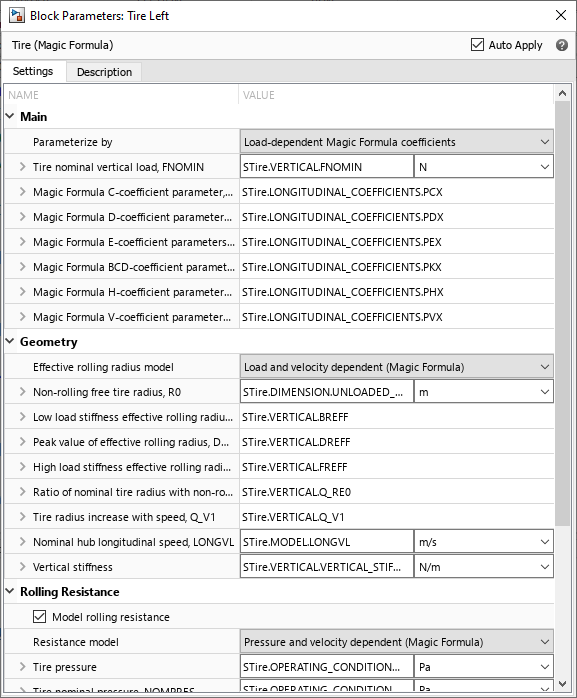Import TIR file to Tire (Magic Formula) block

Since R2022b

## Syntax

``tireParameters = sdlUtility.tirread(fileName)``
``sdlUtility.tirread(block,tireParameters)``

## Description

example

````tireParameters = sdlUtility.tirread(fileName)` returns the tire parameters for the TIR file, `fileName`. The function saves only the information required to parameterize the Tire (Magic Formula) block.```

example

````sdlUtility.tirread(block,tireParameters)` applies the tire parameters from `tireParameters` to the specified Tire (Magic Formula) block.```

## Examples

collapse all

This example shows how to import a TIR file called `sample_tire_245_60_R16.tir` and save the relevant tire parameters as a workspace variable called `STire`. Once you have the parameters saved to the workspace, you can apply the tire parameters to a Tire (Magic Formula) block.

Suppose you have a file named `sample_tire_245_60_R16.tir`. To generate the tire parameters for the file, enter:

`STire = sdlUtility.tirread('sample_tire_245_60_R16.tir')`
```STire = struct with fields: UNITS: [1×1 struct] MODEL: [1×1 struct] DIMENSION: [1×1 struct] OPERATING_CONDITIONS: [1×1 struct] INERTIA: [1×1 struct] ROLLING_COEFFICIENTS: [1×1 struct] VERTICAL: [1×1 struct] STRUCTURAL: [1×1 struct] LONGITUDINAL_COEFFICIENTS: [1×1 struct] LONG_SLIP_RANGE: [1×1 struct] SCALING_COEFFICIENTS: [1×1 struct]```

This example shows how to apply tire parameters in a TIR file to the left tire in the `VehicleWithFourSpeedTransmission` model.

Suppose you have a file named `sample_tire_245_60_R16.tir`. To generate the tire parameters for the file, enter:

`STire = sdlUtility.tirread('sample_tire_245_60_R16.tir')`
```STire = struct with fields: UNITS: [1×1 struct] MODEL: [1×1 struct] DIMENSION: [1×1 struct] OPERATING_CONDITIONS: [1×1 struct] INERTIA: [1×1 struct] ROLLING_COEFFICIENTS: [1×1 struct] VERTICAL: [1×1 struct] STRUCTURAL: [1×1 struct] LONGITUDINAL_COEFFICIENTS: [1×1 struct] LONG_SLIP_RANGE: [1×1 struct] SCALING_COEFFICIENTS: [1×1 struct]```

Determine the path of the tire. The tires are part of the ```Vehicle Body``` subsystem. To parameterize the left tire using the workspace variable `STire`, enter:

`sdlUtility.tirread('VehicleWithFourSpeedTransmission/Vehicle Body/Tire Left', STire);`

Open the Tire Left block. The block uses the parameters in the `STire` structure array.## Input Arguments

collapse all

TIR file name, specified as a character vector or string scalar.

Data Types: `char` | `string`

Tire (Magic Formula) block to parameterize, specified as a character vector or string scalar. You can specify the block path or use the block handle.

Data Types: `char` | `string`

Structure array that contains the tire parameters, specified as a string scalar. The function reduces the parameters to only what the Tire (Magic Formula) block requires.

Data Types: `struct`

## Output Arguments

collapse all

Structure array variable that contains the tire parameters, specified as a structure. The structure contains only the parameters needed to parameterize the Tire (Magic Formula) block.

Data Types: `struct`

## Limitations

• The TIR file must be in the standard format.

• The TIR file must list the units in meters, Newtons, kilograms, and seconds.

• The TIR file must contain all the data needed to parameterize the block.

 Besselink, I. J.M., A. J.C. Schmeitz, and H. B. Pacejka. “An Improved Magic Formula/Swift Tyre Model That Can Handle Inflation Pressure Changes.” Vehicle System Dynamics 48, no. sup1 (December 2010): 337–52. https://doi.org/10.1080/00423111003748088.

 Pacejka, Hans Bastiaan, and Igo Besselink. Tire and Vehicle Dynamics. 3rd edition. Oxford Waltham: Butterworth-Heinemann Elsevier, 2012.